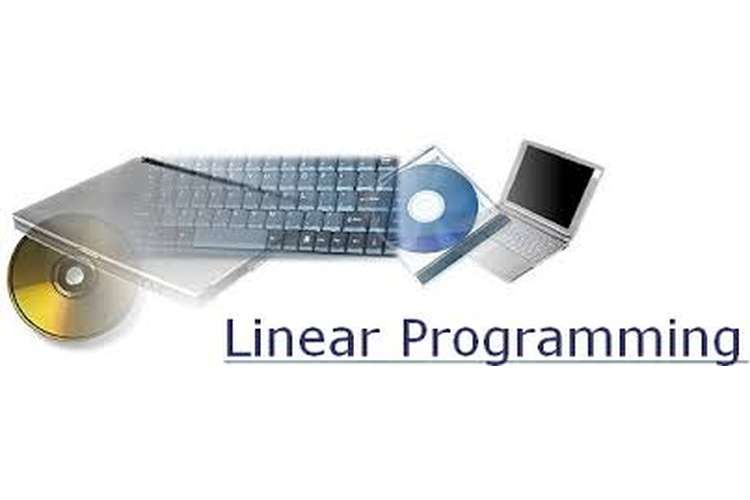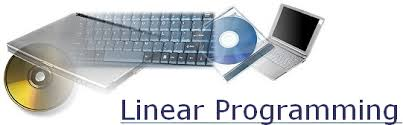# Linear Programming Editing and Proof Reading Services### Linear Programming Editing and Proof Reading Services

This is the solution of linear programming assignment help in which we discuss case study of linear programming and mathematical model• After formulation of given constraints we get
Max Z = 3500x + 5500 y
6x + 8.5 y <= 50 000
4x + 2y <= 25000
Y <= 3800
x,y>= 0
• By graphical methods we are solving the constraints
6x + 8.5 y = 50 000
4x + 2y = 25000
Y <= 3800
• We get following solutions
Z = 2090000 at (0, 3800)
Z = 21875000 at (6250, 0)
Z= 31225000 at (2950, 3800) Maximum profit
Z = 30397700 at (5113.64, 2272.72)
Total door used = 19400 and total door unused = 5600
All the labors hours are used
• We invested \$ 400000 on advertising to increased demand of 25% of silhouette
Now (25/100)x 38000 = 950
So net demand = 4750
Profit Z = 3500x2950 + 3500x 4750 = 36450000
We spent 40000 so net profit = Z = 36410000
so yes the campaign should be undertaken
• Labourtime = 50000+50000x (30/100) = 65000
Max Z = 3500x+ 5500y
6x+8.5y <= 6500
4x+2y <= 25000
Y<=3800
Now solving the above constraints by graphical method we get the solution
(x,y)= (4350, 3800)    solving above constrained graphically we got only one feasible point
Max Z = 36125000 and all doors are used with 6600 used labour hours
• Since in part (c) the total laborhours required to pay is
58400 – 50000 = 8400
So he should pay for 8400 labour hrs.
• Now updating the constraints and solving it by graphical methods we get
Max Z = 3500x+ 5500y
6x+8.5y <= 6500         (1)
4x+2y <= 25000          (2)
Y<=4750                       (3)
Now solving the above constraints by graphical method we get the solution
(x,y)= (3750, 5000)    solving (1) and (2)
(x,y)= (4104.16, 4750) solving (1) and (3)
(x,y)= (3875, 4750)    solving (3) and (2)
No we can conclude that second one is the optimal one because it is satisfying the constraints.
Hence all doors used and 6600 labourhr are unused.
• According to (e)
Z = 414.16x3500+ 4750x 5500= 40489560
Now subtracting some new investment
40489560 – 180000000 = 38689560
SO net profit = 38289560 which is greater than previous one
Hence we go for the condition in (e).
• After formulation
Y< = 2500
Max z = 3500x+ 4950y
X<= 4700
x-2y<= 0
Solving graphically
The feasible optimal solution = (x,y) = (4700, 2350) and the production ratio will be 2:1.
• After formulation
Max z = 3500x + 5500y   (1)
4x+6y <= 50000      (2)
4x+2y<= 25000        (3)
Y<= 3800
Solving (1) and (2)   (875000, -5000)
Solving (3) and (2)    (4550, 3800)
Solving (1) and (3)   (6800, 3800)   max profit
Hence max Z = 258900000
• Since, max profit is less in the case of (h) so, he must not use robot machines.
Considering the conditions in (g) and (i) we got
Number of eagle = 6800
Number of silhouetters = 3800
So the new profit = 22890000
According to the conditions in (j)
Number of doors = 6800x4 + 3800x2 = 34800
Now since the cost of each doors is 800\$
So total cost for the doors = 800x 34800 = 2784000 \$

We can say that Mark does not procure the order for the doors because he is getting loss in this case.